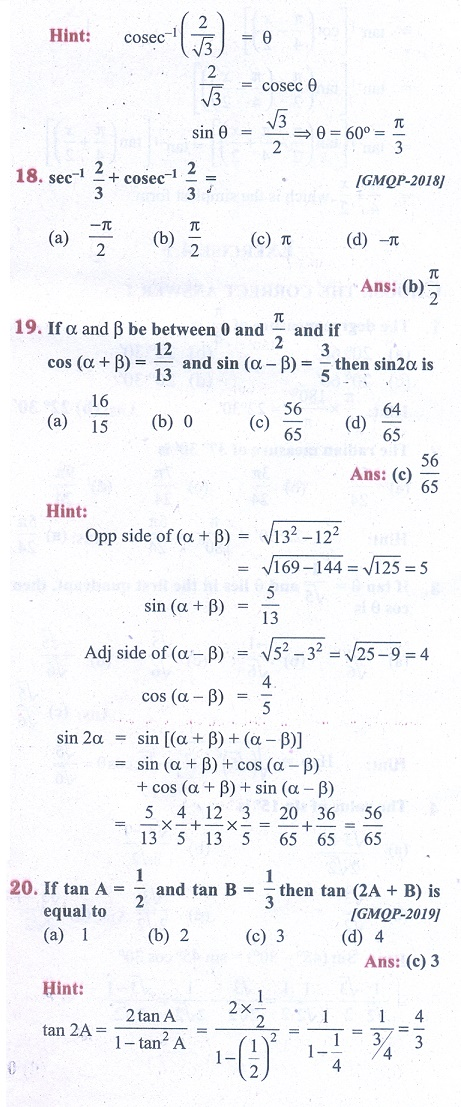Home | | Business Maths 11th std | Exercise 4.5: Choose the correct answer

# Exercise 4.5: Choose the correct answer

Multiple choice questions with answers / choose the correct answer with answers - Business Maths Book back 1 mark questions and answers with solution for Exercise Problems - Mathematics: Trigonometry

Trigonometry - MathematicsTags : Trigonometry | Mathematics , 11th Business Mathematics and Statistics(EMS) : Chapter 4 : Trigonometry
Study Material, Lecturing Notes, Assignment, Reference, Wiki description explanation, brief detail
11th Business Mathematics and Statistics(EMS) : Chapter 4 : Trigonometry : Exercise 4.5: Choose the correct answer | Trigonometry | Mathematics